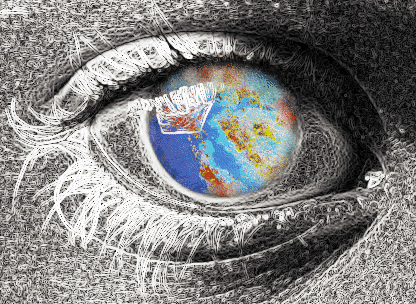## Appendix H. Results of Operators

This appendix presents examples in the following sections of all the operators that give visible results:

For more information on using these operators and what effect they have on image data, see Chapter 4, “Operating on an Image.” More specific information on how to apply each operator is located in its header file and in its reference page.

Original, unprocessed images are presented where necessary. Some images combine two original images. These images are either reversed copies of the same image or two extremely similar images.

## Color Conversion

Figure H-1. ilFalseColorImg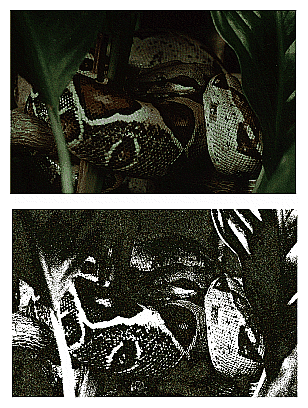Figure H-2. ilGrayImg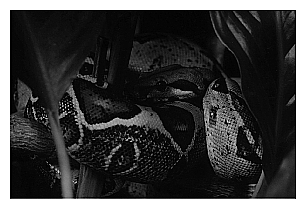## Arithmetic and Logical Transformations

Figure H-3. Original Image and Flipped Image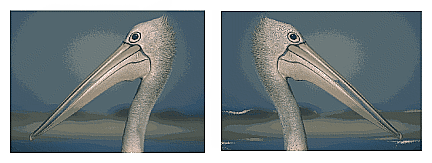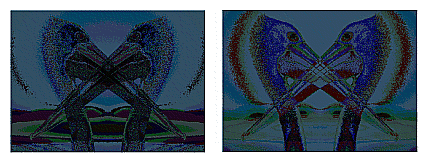Figure H-5. ilDivImg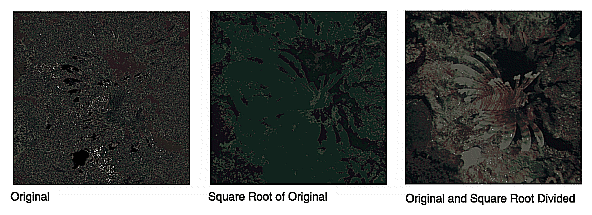Figure H-6. ilExpImg and ilInvertImg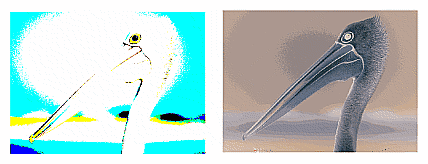Figure H-7. ilLogImg and ilMaxImg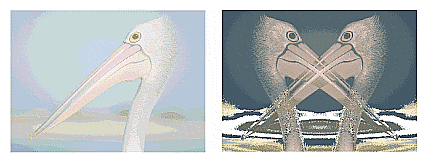Figure H-8. ilMinImg and ilMultiplyImg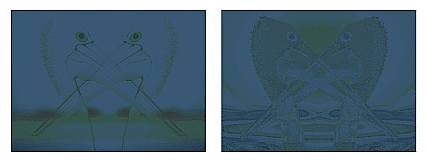Figure H-9. ilNegImg and ilOrImg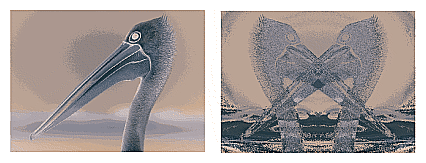Figure H-10. ilPowerImg and ilSqRootImg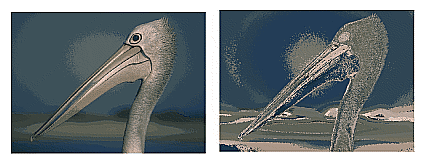Figure H-11. ilSquareImg and ilSubtractImg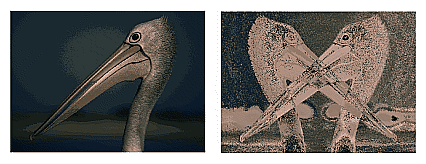Figure H-12. ilXorImg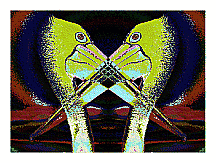## Geometric Transformations

Figure H-13. Original and ilRotZoomImg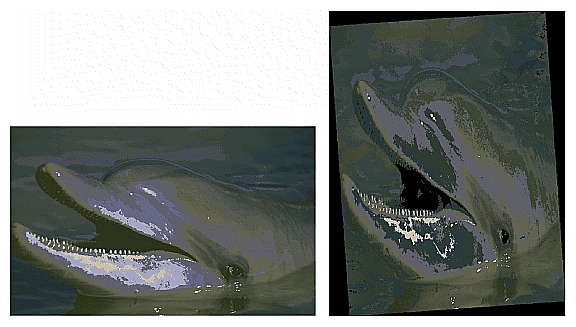Figure H-14. ilWarpImg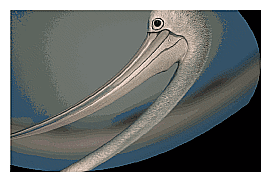## Spatial Domain Transformations

Figure H-15. Original, ilBlurImg and ilGBlurImg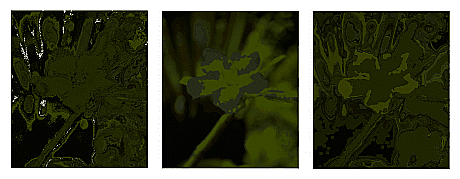Figure H-16. ilDilateImg, ilErodeImg, and ilMaxFltImg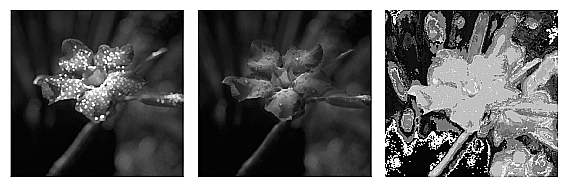Figure H-17. ilMedFltImg, ilMinFltImg, and ilSharpenImg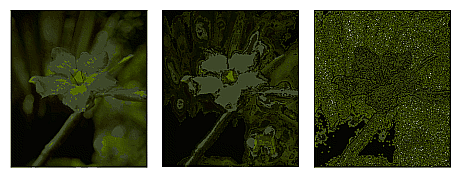## Edge Detection

Figure H-18. ilCompassImg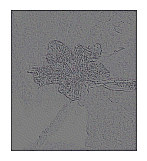Figure H-19. ilLaplaceImg (original and filtered image)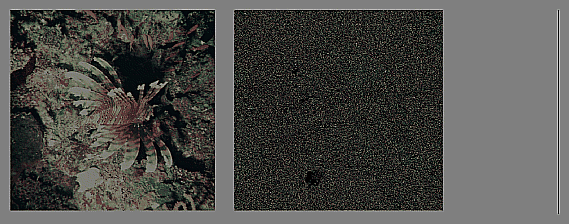Figure H-20. ilRobertsImg (original and filtered image)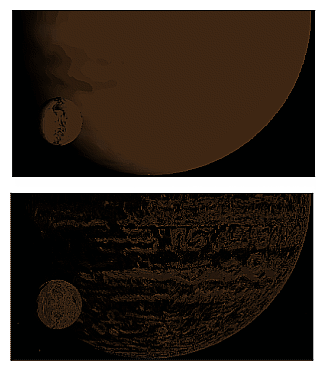Figure H-21. ilSobelImg (original and filtered image)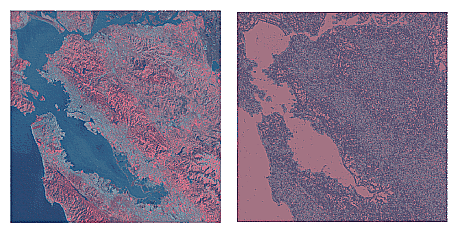## Frequency Domain Transformations

The frequency domain transformations are of limited interest as illustrations. For the purposes of this appendix, one example is shown. In the example, an original image is presented along with its appearance in the frequency, or Fourier domain, and the filtered resultant image is shown in both the spatial and frequency domains.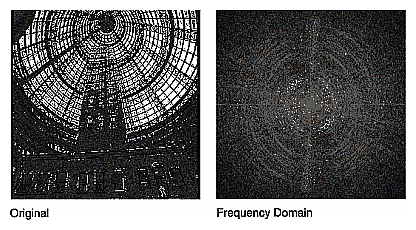Figure H-22. ilFGaussFiltImg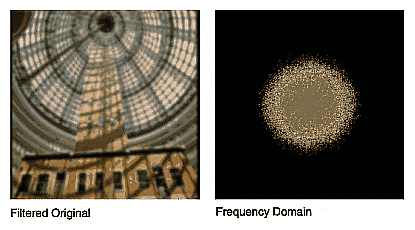Figure H-23. ilHistEqImg (filtered image and histogram)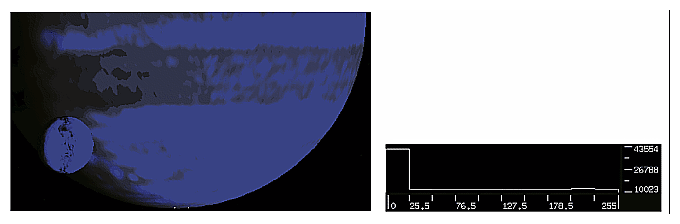Figure H-24. ilHistNormImg (filtered image and histogram)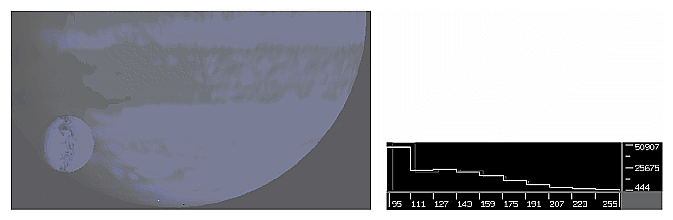Figure H-25. ilHistScaleImg (filtered image and histogram)Figure H-26. ilLutImg (original, filtered image, and LUT editor)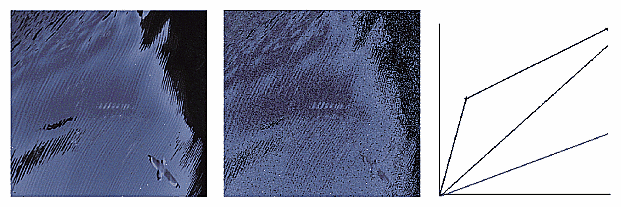Figure H-27. ilThreshImg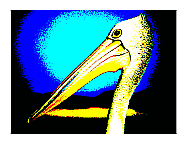## Combining Images

Figure H-28. Originals and Original Mask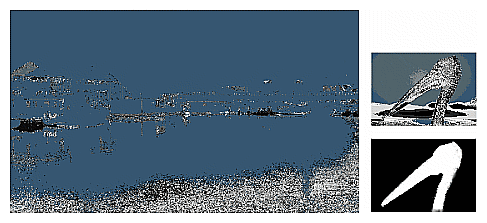Figure H-29. ilBlendImg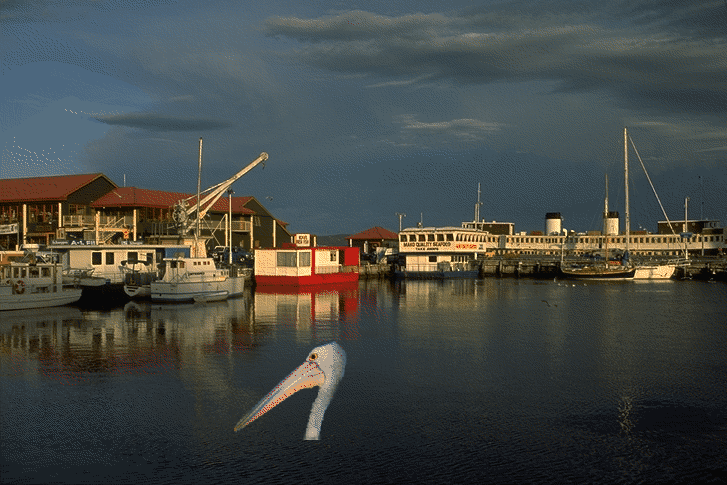Figure H-30. ilCombineImg